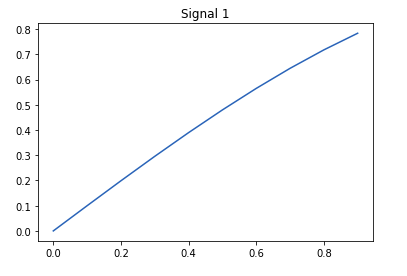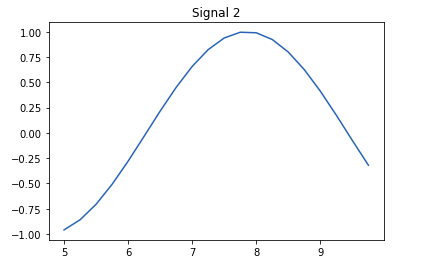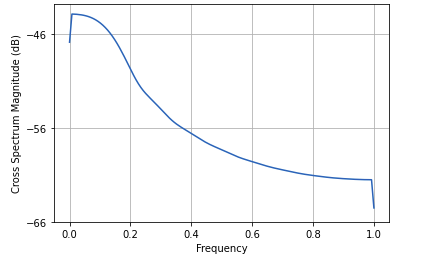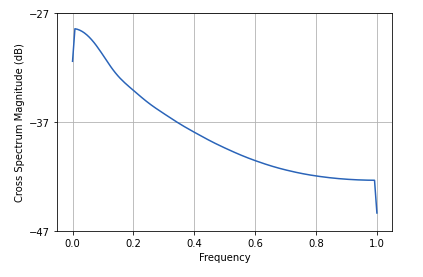Open In App

# Plotting cross-spectral density in Python using Matplotlib

Matplotlib is a comprehensive library consisting of modules that are used for Data Visualization just like MATLAB. Pyplot is a further module which makes functions and methods executable.

## Plotting Cross-Spectral Density

The cross-spectral density compares two signals, each from different source taking into account both amplitude and phase difference of the two signals. In Python, this function is carried out using the Pyplot module’s method matplotlib.pyplot.csd()
Syntax:

`matplotlib.pyplot.csd(x, y)`

Here, x and y are 1-D arrays or a sequence having the data.
Let us take two signals and plot their CSD:

1. Signal 1 has time period from 0 to 1 second and 0.1 radian phase angle with frequency being calculated using sin() function.
2. Similarly, Signal 2 has time period from 5 to 10 seconds and 0.25 radians phase angle.
3. Taking these two signals, we plot their cross spectral density.

Example 1: Plotting Signal 1

## python3

 `import` `numpy as np``import` `matplotlib.pyplot as plt`  `time ``=` `np.arange(``0``, ``1``, ``0.1``)``amp ``=` `np.sin(time)` `plt.plot(time, amp)``plt.title("Signal ``1``")` `plt.show()`

Output:Example 2: Plotting Signal 2

## Python3

 `import` `numpy as np``import` `matplotlib.pyplot as plt`  `t ``=` `np.arange(``5``, ``10``, ``0.25``)``ampl ``=` `np.sin(t)` `plt.plot(t, ampl)``plt.title("Signal ``2``")` `plt.show()`

Output:Example 3: Plotting the cross-spectral density

## Python3

 `import` `numpy as np``import` `matplotlib.pyplot as plt`  `# Signal 1``time ``=` `np.arange(``0``, ``1``, ``0.1``)``amp ``=` `np.sin(time)` `# Signal 2``t ``=` `np.arange(``5``, ``10``, ``0.25``)``ampl ``=` `np.sin(t)` `# Cross-spectral density``plt.csd(amp, ampl)` `plt.show()`

Output:Example 4: Using discrete lists or arrays

## Python3

 `import` `numpy as np``import` `matplotlib.pyplot as plt`  `a ``=` `np.arange(``5``)``b ``=` `np.arange(``10``, ``30``)` `plt.csd(a, b)``plt.show()`

Output: